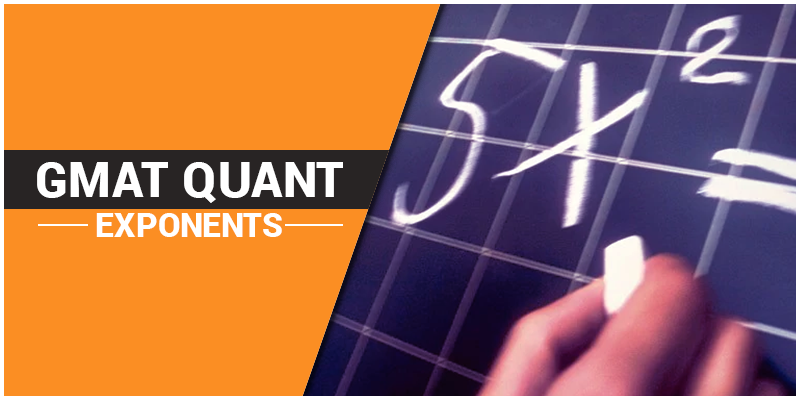# GMAT Quant: Algebra – ExponentsExponents are shorthand for repeated multiplication of the same thing by itself. For Example – $5 \times 5 \times 5 = 5^{3} = 125$

## Exponents Rules and Properties

Zero rules

$a^{0} = 1$

$0^{n} = 0 ; \; for \; n > 0$

One rules

$a^{1} = a$

Minus one rule

$(-1)^{n} = \left\{\begin{matrix} 1 & ,n\; even\\ -1 & ,n\; odd \end{matrix}\right.$

Power rules

$b^{n^{m}} = b^{(n^{m})}$

$\sqrt[m]{(b^{n})} = (b)^{\frac{n}{m}}$

$b^{\frac{1}{n}} = \sqrt[n]{b}$

Product rules

$a^{n} . a^{m} = a^{n+m}$

$a^{n} . b^{n} = (a.b)^{n}$

Quotient rules

$\frac{a^{n}}{a^{m}}= a^{n-m}$

$\frac{a^{n}}{b^{n}}= \left ( \frac{a}{b} \right )^{n}$

Negative exponents

$b^{-n} = \frac{1}{b^{n}}$

## Roots & Powers

Some basic formulae of Roots are –

$\sqrt[n]{a} = a^{\frac{1}{n}}$

$\sqrt[n]{ab} = \sqrt[n]{a}\sqrt[n]{b}$

$\sqrt[n]{\frac{a}{b}} = \frac{\sqrt[n]{a}}{\sqrt[n]{b}}$

$(\sqrt[n]{a})^{n} = a$

Few Solved Examples

Example 1 – If $5^{3x} = 5^{(7x -2)}$; Then x = ?
Solution – As both the sides have same base,
Therefore, $3x = 7x -2$ $2 = 4x$ $x = \frac{1}{2}$

Example 2 – Solve for t, where $4^{t^{2}} = 4^{6-t}$ Solution – We can write the equation as $t^{2} = 6-t$ $t^{2} + t -6 = 0$ $(t +3)(t-2) = 0$ $\Rightarrow t = -3, t = 2$

Example 3 – z = ?, If, $3^{z} = 9^{z + 5}$ $3^{z} = 3^{2}^{(z + 5)}$ Therefore, $z = 2(z + 5)$ $z = 2z + 10$ $z = – 10$

Example 4 – Find x; $4^{5 – 9x} = \frac{1}{8^{(x -2)}}$ $(2^2)^{5 – 9x} = \frac{1}{2^3^{(x -2)}}$ $As \; \frac{1}{a^{n}} = a^{-n}$ Hence, $2^{2}^{(5 – 9x)} = 2^{-3}^{(x – 2)}$ $2(5 – 9x) = -3(x – 2)$ $10 – 18x = -3x + 6$ $4 = 15x$ $x = \frac{4}{15}$

Hope these formulas and examples assist you in brushing up what you had learned in your mathematics classes during your school. Afterall, GMAT question pattern is entirely based on the basics. Click to know more on GMAT Syllabus and GMAT Exam Pattern.

BYJU’S will be glad to help you in your GMAT preparation journey. You can ask for any assistance related to GMAT and MBA from us by calling us at +918884544444. You can write to us at gmat@byjus.com.